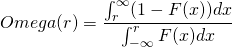# Omega ratio

The omega ratio is a risk-return measure, like the Sharpe ratio, helps investors to assess the attractiveness of a hedge fund, mutual fund, or individual security. But unlike the Sharpe ratio, which only takes into account the volatility, the omega ratio also considers the so-called higher moments of the distribution. The omega measure is often used in the context of alternative investments (e.g. hedge funds) where the manager promises absolute performance. In such cases, however, the return distribution can be asymmetric, with considerable tail risk or negative skewness. The sharpe ratio is not able to capture these features of the return distribution.

## Omega ratio definition

The omega ratio was first proposed by Keating and & Shadwick (2002). Formally, the ratio is defined as

the probability-weighted ratio of gains versus losses for a given minimum acceptable return.

To calculate the omega ratio, we make use of the ‘cumulative distribution function’ of the returns of the security we want to analyze. In particular, we calculate the area under the chart or integral around a certain threshold return level. As in the above definition, we refer to this threshold minimum return level as the minimum acceptable return or MAR for short. The integral below the MAR measures the ‘weight’ of the losing part of a strategy (sum of the losses). The integral above the MAR measures the ‘weight’ of the gains. The ratio between the two indicates by how much the gains part outperforms the losing part.

The big advantage of using the area or integral is that we take into consideration the entire distribution of the returns. This is different from the Sharpe ratio, which only considers the mean and standard deviation of returns. In contrast to the Sharpe ratio, the omega looks at the entire distribution, thereby considering, amongst others, the kurtosis and skewness of the distribution. In fact, the omega ratio considers all moments. This is important, for example, when the returns from a strategy are asymmetric.

## Omega ratio formula

The above definition may sound a bit abstract to some. Therefore, let’s turn to the formula of the omega ratio:where F equals the cumulative distribution function of the returns and r is the minimum acceptable return (MAR) that defines what we consider a gain or a loss. Hence, the MAR can be a number different from zero. For those unfamiliar with integrals, we implement the approach using Excel, which will help to make the above concepts clear to everyone.

## Omega ratio example

Finally, lets discuss how one can calculate the omega ratio using spreadsheet software such as Excel. In the file below, we calculate the omega ratio for a given Sharpe ratio using simulated annual return data. The example that implements a simple calculator can be downloaded here.

## Summary

We discussed the omega ratio, a measure that takes into consideration the entire return distribution when assessing performance. The ratio is especially valuable when the returns of an investment are not normally distributed. This is the case for most investments in practice. In the case of hedge fund performance, the use of this ratio is becoming  widespread.

### Omega ratio calculator

Want to have an implementation in Excel? Download the Excel file: Omega ratio excel example